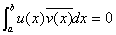、希尔伯特-施密特的理论

[具有对称核的Fr方程的性质]  如果在实核中交换它的变量时，它本身的值不变，这个核就叫做对称核。

[希尔伯特-施密特定理]  Φ为一平方可积函数，则形如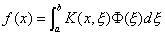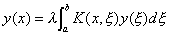[a,b]上的特征函数y1(x), y2(x),L的线性组合表达，如果特征函数有无穷多个，那末所得的无穷级数在区间[a,b]上绝对且一致收敛。

[施密特公式]  考虑非齐次第二类Fr方程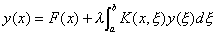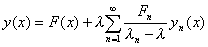λλn ，即λ不是特征值）                 (1)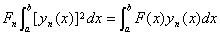n=1,2,L                     (2)

[核的展开定理]  一个对称核K(x,x)可展开为级数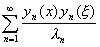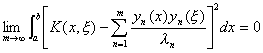[具有非对称核的积分方程]  设核K(x,x )不是对称的，但可表为如下形式

K(x,x )=r(x )G(x,x )

对应于不同特征值l ml n的两个特征函数ym(x)yn(x)[a,b]上关于权函数r(x)是正交的，即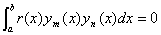K(x,x )的特征值都是实数。

若非齐次第二类Fr方程有一个解，则这个解由(1)给出，并以权函数r(x)去乘(2)式两边所包含的被积函数。

[具有埃尔米特核的积分方程]  设核K(x,x )为一复核，如果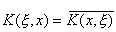对应于不同特征值l ml n的两个特征函数ym(x)yn(x)[a,b]上是按埃尔米特意义正交的：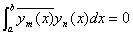[a,b]上与埃尔米特核相联系的特征值都是实数。

设特征函数按埃尔米特意义是标准化的：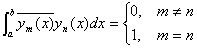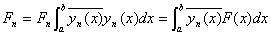n=1,2,L

[具有反对称核的积分方程]  K(x,x )满足条件

K(x ,x)=-K(x,x )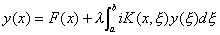[伴随核与自伴随核]  u(x)是一复核K(x,x )(它不一定是埃尔米特核)对应于特征值l 的一个特征函数，v(x)是核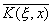对应于特征值m 的一个特征函数，若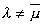，则